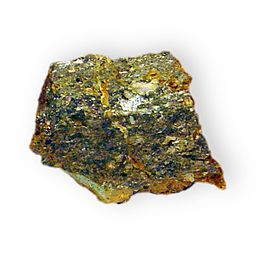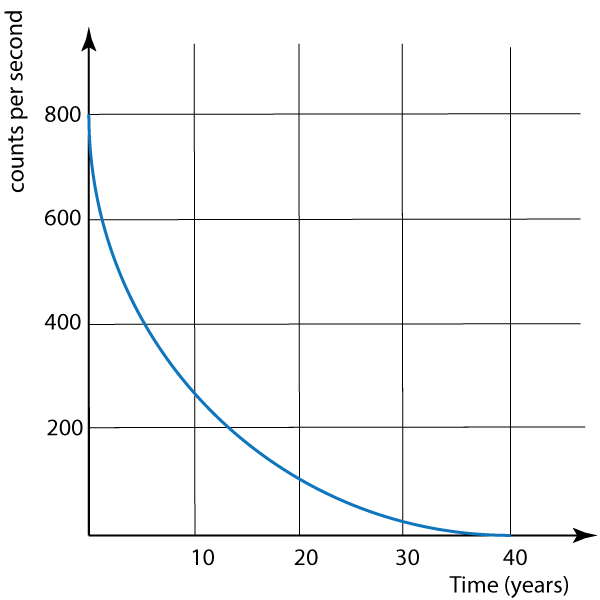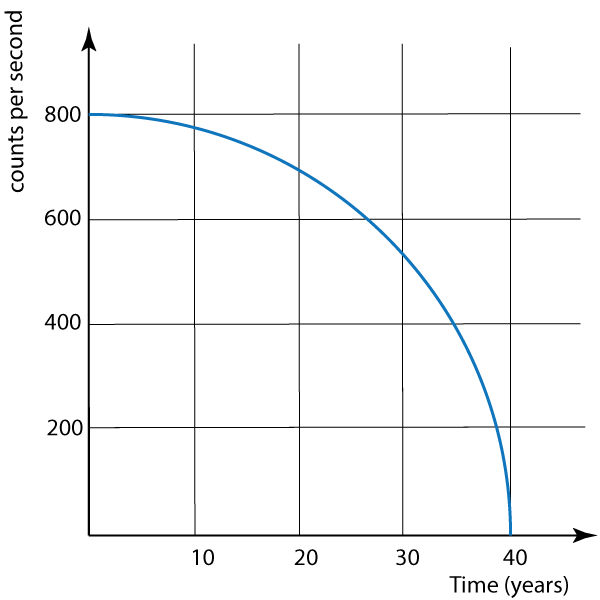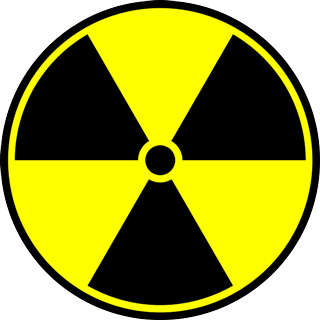µ GCSE | Half Life

10 quick questions - for GCSE and iGCSE

10 minutes maximum! (can you do it in 5?)

1. Which of these is the best description of the 'activity' of a radioactive rock?

• A. The total number of radioactive atoms that have decayed.
• B. The total number of radioactive atoms remaining undecayed in the rock.
• C. The number of gamma rays emmited per second.
• D. The number of radioactive atoms that have decayed per second.

2. What are the units used to measure the Activity of a sample of rock?

• A. coulombs (C)
• B. becquerels (Bq)
• C. joules (J)
• D. watts (W)Q3-6.

The graphs below show 4 ways the count rate from a radioactive isotope could change:

 A BC D3. Which of the above graphs shows the correct shape for radioactive decay?

4. Using the correct graph, what is the half life of the sample?

• A. 5 years
• B. 10 years
• C. 20 years
• D. 40 years

5. What is the activity of the sample after 2 half lifes?

• A. 800 counts per second
• B. 400 counts per second
• C. 200 counts per second
• D. 0 counts per second

6. Use the correct graph to estimate the count rate after 30 years.

• A. 100 counts per second
• B. 200 counts per second
• C. 25 counts per second
• D. 550 counts per second

Q7-10: An isotope has a half life of 8 hours.

7. How much of the sample will remain after 24 hours?

• A. 12
• B. 13
• C. 14
• D. 188. If the mass of the isotope is 12 g of pure radioactive isotope, how many grams remains after 16 hours?

• A. 0.75 g
• B. 3 g
• C. 4 g
• D. 6 g

9. What has happened to the rest of the mass of the isotope?

• A. All converted to radiation
• B. All converted to heat energy.
• C. Converted into a new element.
• D. remained the same isotope but evaporated.

10. Here are three suggestions for safe use for the isotope:

Which of these are realistic and sensible suggestions?

• A. II only
• B. I and II
• C. II and III
• D. All three

I. Do not handle directly - use tongs.

II. Store in a thick lead or steel box.

III. Do not look directly at the isotope whilst handling it.